Elementary Algebra 2e

# 4.1Use the Rectangular Coordinate System

Elementary Algebra 2e4.1 Use the Rectangular Coordinate System

### Learning Objectives

By the end of this section, you will be able to:

• Plot points in a rectangular coordinate system
• Verify solutions to an equation in two variables
• Complete a table of solutions to a linear equation
• Find solutions to a linear equation in two variables

### Be Prepared 4.1

Before you get started, take this readiness quiz.

Evaluate $x+3x+3$ when $x=−1x=−1$.
If you missed this problem, review Example 1.54.

### Be Prepared 4.2

Evaluate $2x−5y2x−5y$ when $x=3x=3$ and $y=−2.y=−2.$
If you missed this problem, review Example 1.55.

### Be Prepared 4.3

Solve for $yy$: $40−4y=2040−4y=20$.
If you missed this problem, review Example 2.27.

### Plot Points on a Rectangular Coordinate System

Just like maps use a grid system to identify locations, a grid system is used in algebra to show a relationship between two variables in a rectangular coordinate system. The rectangular coordinate system is also called the xy-plane or the ‘coordinate plane’.

The horizontal number line is called the x-axis. The vertical number line is called the y-axis. The x-axis and the y-axis together form the rectangular coordinate system. These axes divide a plane into four regions, called quadrants. The quadrants are identified by Roman numerals, beginning on the upper right and proceeding counterclockwise. See Figure 4.2.

In the rectangular coordinate system, every point is represented by an ordered pair. The first number in the ordered pair is the x-coordinate of the point, and the second number is the y-coordinate of the point.

### Ordered Pair

An ordered pair, $(x,y)(x,y)$, gives the coordinates of a point in a rectangular coordinate system.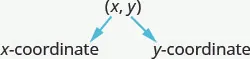The first number is the x-coordinate.

The second number is the y-coordinate.

The phrase ‘ordered pair’ means the order is important. What is the ordered pair of the point where the axes cross? At that point both coordinates are zero, so its ordered pair is $(0,0)(0,0)$. The point $(0,0)(0,0)$ has a special name. It is called the origin.

### The Origin

The point $(0,0)(0,0)$ is called the origin. It is the point where the x-axis and y-axis intersect.

We use the coordinates to locate a point on the xy-plane. Let’s plot the point $(1,3)(1,3)$ as an example. First, locate 1 on the x-axis and lightly sketch a vertical line through $x=1x=1$. Then, locate 3 on the y-axis and sketch a horizontal line through $y=3y=3$. Now, find the point where these two lines meet—that is the point with coordinates $(1,3)(1,3)$.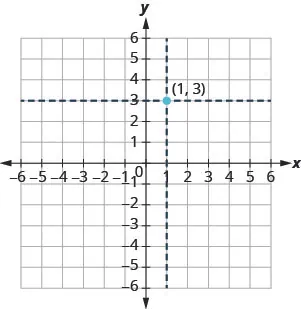Notice that the vertical line through $x=1x=1$ and the horizontal line through $y=3y=3$ are not part of the graph. We just used them to help us locate the point $(1,3)(1,3)$.

### Example 4.1

Plot each point in the rectangular coordinate system and identify the quadrant in which the point is located:

$(−5,4)(−5,4)$ $(−3,−4)(−3,−4)$ $(2,−3)(2,−3)$ $(−2,3)(−2,3)$ $(3,52)(3,52)$.

### Try It 4.1

Plot each point in a rectangular coordinate system and identify the quadrant in which the point is located:

$(−2,1)(−2,1)$ $(−3,−1)(−3,−1)$ $(4,−4)(4,−4)$ $(−4,4)(−4,4)$ $(−4,32)(−4,32)$.

### Try It 4.2

Plot each point in a rectangular coordinate system and identify the quadrant in which the point is located:

$(−4,1)(−4,1)$ $(−2,3)(−2,3)$ $(2,−5)(2,−5)$ $(−2,5)(−2,5)$ $(−3,52)(−3,52)$.

How do the signs affect the location of the points? You may have noticed some patterns as you graphed the points in the previous example.

For the point in Figure 4.3 in Quadrant IV, what do you notice about the signs of the coordinates? What about the signs of the coordinates of points in the third quadrant? The second quadrant? The first quadrant?

Can you tell just by looking at the coordinates in which quadrant the point $(−2,5)(−2,5)$ is located? In which quadrant is $(2,−5)(2,−5)$ located?

We can summarize sign patterns of the quadrants in this way.

$Quadrant IQuadrant IIQuadrant IIIQuadrant IV(x,y)(x,y)(x,y)(x,y)(+,+)(−,+)(−,−)(+,−)Quadrant IQuadrant IIQuadrant IIIQuadrant IV(x,y)(x,y)(x,y)(x,y)(+,+)(−,+)(−,−)(+,−)$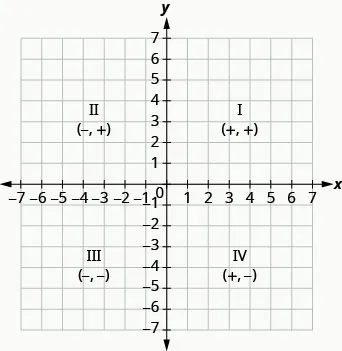What if one coordinate is zero as shown in Figure 4.4? Where is the point $(0,4)(0,4)$ located? Where is the point $(−2,0)(−2,0)$ located?

Figure 4.4

The point $(0,4)(0,4)$ is on the y-axis and the point $(−2,0)(−2,0)$ is on the x-axis.

### Points on the Axes

Points with a y-coordinate equal to 0 are on the x-axis, and have coordinates $(a,0)(a,0)$.

Points with an x-coordinate equal to 0 are on the y-axis, and have coordinates $(0,b)(0,b)$.

### Example 4.2

Plot each point:

$(0,5)(0,5)$ $(4,0)(4,0)$ $(−3,0)(−3,0)$ $(0,0)(0,0)$ $(0,−1)(0,−1)$.

### Try It 4.3

Plot each point:

$(4,0)(4,0)$ $(−2,0)(−2,0)$ $(0,0)(0,0)$ $(0,2)(0,2)$ $(0,−3)(0,−3)$.

### Try It 4.4

Plot each point:

$(−5,0)(−5,0)$ $(3,0)(3,0)$ $(0,0)(0,0)$ $(0,−1)(0,−1)$ $(0,4)(0,4)$.

In algebra, being able to identify the coordinates of a point shown on a graph is just as important as being able to plot points. To identify the x-coordinate of a point on a graph, read the number on the x-axis directly above or below the point. To identify the y-coordinate of a point, read the number on the y-axis directly to the left or right of the point. Remember, when you write the ordered pair use the correct order, $(x,y)(x,y)$.

### Example 4.3

Name the ordered pair of each point shown in the rectangular coordinate system.### Try It 4.5

Name the ordered pair of each point shown in the rectangular coordinate system.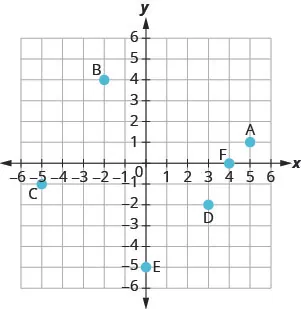### Try It 4.6

Name the ordered pair of each point shown in the rectangular coordinate system.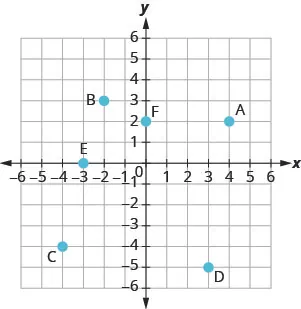### Verify Solutions to an Equation in Two Variables

Up to now, all the equations you have solved were equations with just one variable. In almost every case, when you solved the equation you got exactly one solution. The process of solving an equation ended with a statement like $x=4x=4$. (Then, you checked the solution by substituting back into the equation.)

Here’s an example of an equation in one variable, and its one solution.

$3x+5=173x=12x=43x+5=173x=12x=4$

But equations can have more than one variable. Equations with two variables may be of the form $Ax+By=CAx+By=C$. Equations of this form are called linear equations in two variables.

### Linear Equation

An equation of the form $Ax+By=CAx+By=C$, where $AA$ and $BB$ are not both zero, is called a linear equation in two variables.

Notice the word line in linear. Here is an example of a linear equation in two variables, $xx$ and $yy$.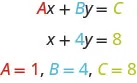The equation $y=−3x+5y=−3x+5$ is also a linear equation. But it does not appear to be in the form $Ax+By=CAx+By=C$. We can use the Addition Property of Equality and rewrite it in $Ax+By=CAx+By=C$ form.

 $y=−3x+5y=−3x+5$ Add to both sides. $y+3x=−3x+5+3xy+3x=−3x+5+3x$ Simplify. $y+3x=5y+3x=5$ Use the Commutative Property to put it in $Ax+By=CAx+By=C$ form. $3x+y=53x+y=5$
Table 4.1

By rewriting $y=−3x+5y=−3x+5$ as $3x+y=53x+y=5$, we can easily see that it is a linear equation in two variables because it is of the form $Ax+By=CAx+By=C$. When an equation is in the form $Ax+By=CAx+By=C$, we say it is in standard form.

### Standard Form of Linear Equation

A linear equation is in standard form when it is written $Ax+By=CAx+By=C$.

Most people prefer to have $AA$, $BB$, and $CC$ be integers and $A≥0A≥0$ when writing a linear equation in standard form, although it is not strictly necessary.

Linear equations have infinitely many solutions. For every number that is substituted for $xx$ there is a corresponding $yy$ value. This pair of values is a solution to the linear equation and is represented by the ordered pair $(x,y)(x,y)$. When we substitute these values of $xx$ and $yy$ into the equation, the result is a true statement, because the value on the left side is equal to the value on the right side.

### Solution of a Linear Equation in Two Variables

An ordered pair $(x,y)(x,y)$ is a solution of the linear equation $Ax+By=CAx+By=C$, if the equation is a true statement when the x- and y-values of the ordered pair are substituted into the equation.

### Example 4.4

Determine which ordered pairs are solutions to the equation $x+4y=8x+4y=8$.

$(0,2)(0,2)$ $(2,−4)(2,−4)$ $(−4,3)(−4,3)$

### Try It 4.7

Which of the following ordered pairs are solutions to $2x+3y=62x+3y=6$?

$(3,0)(3,0)$ $(2,0)(2,0)$ $(6,−2)(6,−2)$

### Try It 4.8

Which of the following ordered pairs are solutions to the equation $4x−y=84x−y=8$?

$(0,8)(0,8)$ $(2,0)(2,0)$ $(1,−4)(1,−4)$

### Example 4.5

Which of the following ordered pairs are solutions to the equation $y=5x−1y=5x−1$?

$(0,−1)(0,−1)$ $(1,4)(1,4)$ $(−2,−7)(−2,−7)$

### Try It 4.9

Which of the following ordered pairs are solutions to the equation $y=4x−3y=4x−3$?

$(0,3)(0,3)$ $(1,1)(1,1)$ $(−1,−1)(−1,−1)$

### Try It 4.10

Which of the following ordered pairs are solutions to the equation $y=−2x+6y=−2x+6$?

$(0,6)(0,6)$ $(1,4)(1,4)$ $(−2,−2)(−2,−2)$

### Complete a Table of Solutions to a Linear Equation in Two Variables

In the examples above, we substituted the x- and y-values of a given ordered pair to determine whether or not it was a solution to a linear equation. But how do you find the ordered pairs if they are not given? It’s easier than you might think—you can just pick a value for $xx$ and then solve the equation for $yy$. Or, pick a value for $yy$ and then solve for $xx$.

We’ll start by looking at the solutions to the equation $y=5x−1y=5x−1$ that we found in Example 4.5. We can summarize this information in a table of solutions, as shown in Table 4.2.

 $y=5x−1y=5x−1$ $xx$ $yy$ $(x,y)(x,y)$ 0 $−1−1$ $(0,−1)(0,−1)$ 1 4 $(1,4)(1,4)$
Table 4.2

To find a third solution, we’ll let $x=2x=2$ and solve for $yy$.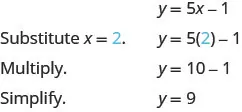The ordered pair $(2,9)(2,9)$ is a solution to $y=5x−1y=5x−1$. We will add it to Table 4.3.

 $y=5x−1y=5x−1$ $xx$ $yy$ $(x,y)(x,y)$ 0 $−1−1$ $(0,−1)(0,−1)$ 1 4 $(1,4)(1,4)$ 2 9 $(2,9)(2,9)$
Table 4.3

We can find more solutions to the equation by substituting in any value of $xx$ or any value of $yy$ and solving the resulting equation to get another ordered pair that is a solution. There are infinitely many solutions of this equation.

### Example 4.6

Complete Table 4.4 to find three solutions to the equation $y=4x−2y=4x−2$.

 $y=4x−2y=4x−2$ $xx$ $yy$ $(x,y)(x,y)$ 0 $−1−1$ 2
Table 4.4

### Try It 4.11

Complete the table to find three solutions to this equation: $y=3x−1y=3x−1$.

 $y=3x−1y=3x−1$ $xx$ $yy$ $(x,y)(x,y)$ 0 $−1−1$ 2

### Try It 4.12

Complete the table to find three solutions to this equation: $y=6x+1y=6x+1$.

 $y=6x+1y=6x+1$ $xx$ $yy$ $(x,y)(x,y)$ 0 1 $−2−2$

### Example 4.7

Complete Table 4.6 to find three solutions to the equation $5x−4y=205x−4y=20$.

 $5x−4y=205x−4y=20$ $xx$ $yy$ $(x,y)(x,y)$ 0 0 5
Table 4.6

### Try It 4.13

Complete the table to find three solutions to this equation: $2x−5y=202x−5y=20$.

 $2x−5y=202x−5y=20$ $xx$ $yy$ $(x,y)(x,y)$ 0 0 $−5−5$

### Try It 4.14

Complete the table to find three solutions to this equation: $3x−4y=123x−4y=12$.

 $3x−4y=123x−4y=12$ $xx$ $yy$ $(x,y)(x,y)$ 0 0 $−4−4$

### Find Solutions to a Linear Equation

To find a solution to a linear equation, you really can pick any number you want to substitute into the equation for $xx$ or $y.y.$ But since you’ll need to use that number to solve for the other variable it’s a good idea to choose a number that’s easy to work with.

When the equation is in y-form, with the y by itself on one side of the equation, it is usually easier to choose values of $xx$ and then solve for $yy$.

### Example 4.8

Find three solutions to the equation $y=−3x+2y=−3x+2$.

### Try It 4.15

Find three solutions to this equation: $y=−2x+3y=−2x+3$.

### Try It 4.16

Find three solutions to this equation: $y=−4x+1y=−4x+1$.

We have seen how using zero as one value of $xx$ makes finding the value of $yy$ easy. When an equation is in standard form, with both the $xx$ and $yy$ on the same side of the equation, it is usually easier to first find one solution when $x=0x=0$ find a second solution when $y=0y=0$, and then find a third solution.

### Example 4.9

Find three solutions to the equation $3x+2y=63x+2y=6$.

### Try It 4.17

Find three solutions to the equation $2x+3y=62x+3y=6$.

### Try It 4.18

Find three solutions to the equation $4x+2y=84x+2y=8$.

### Section 4.1 Exercises

#### Practice Makes Perfect

Plot Points in a Rectangular Coordinate System

In the following exercises, plot each point in a rectangular coordinate system and identify the quadrant in which the point is located.

1.
1. $(−4,2)(−4,2)$
2. $(−1,−2)(−1,−2)$
3. $(3,−5)(3,−5)$
4. $(−3,5)(−3,5)$
$(53,2)(53,2)$
2.
1. $(−2,−3)(−2,−3)$
2. $(3,−3)(3,−3)$
3. $(−4,1)(−4,1)$
4. $(4,−1)(4,−1)$
5. $(32,1)(32,1)$
3.
1. $(3,−1)(3,−1)$
2. $(−3,1)(−3,1)$
3. $(−2,2)(−2,2)$
4. $(−4,−3)(−4,−3)$
5. $(1,145)(1,145)$
4.
1. $(−1,1)(−1,1)$
2. $(−2,−1)(−2,−1)$
3. $(2,1)(2,1)$
4. $(1,−4)(1,−4)$
5. $(3,72)(3,72)$

In the following exercises, plot each point in a rectangular coordinate system.

5.
1. $(−2,0)(−2,0)$
2. $(−3,0)(−3,0)$
3. $(0,0)(0,0)$
4. $(0,4)(0,4)$
5. $(0,2)(0,2)$
6.
1. $(0,1)(0,1)$
2. $(0,−4)(0,−4)$
3. $(−1,0)(−1,0)$
4. $(0,0)(0,0)$
5. $(5,0)(5,0)$
7.
1. $(0,0)(0,0)$
2. $(0,−3)(0,−3)$
3. $(−4,0)(−4,0)$
4. $(1,0)(1,0)$
5. $(0,−2)(0,−2)$
8.
1. $(−3,0)(−3,0)$
2. $(0,5)(0,5)$
3. $(0,−2)(0,−2)$
4. $(2,0)(2,0)$
5. $(0,0)(0,0)$

In the following exercises, name the ordered pair of each point shown in the rectangular coordinate system.

9.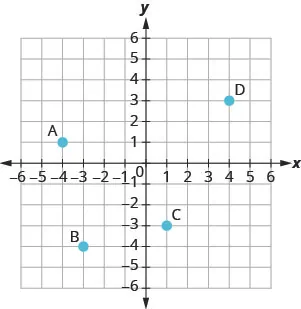10.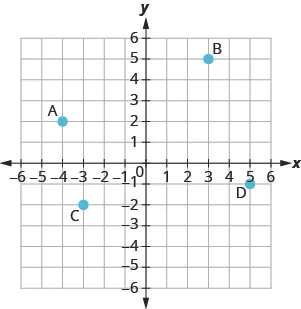11.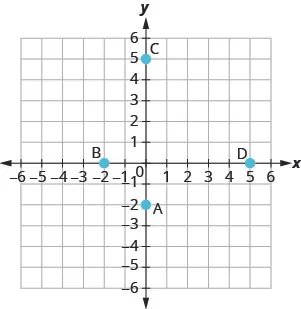12.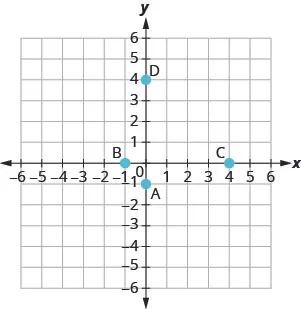Verify Solutions to an Equation in Two Variables

In the following exercises, which ordered pairs are solutions to the given equations?

13.

$2 x + y = 6 2 x + y = 6$

1. $(1,4)(1,4)$
2. $(3,0)(3,0)$
3. $(2,3)(2,3)$
14.

$x + 3 y = 9 x + 3 y = 9$

1. $(0,3)(0,3)$
2. $(6,1)(6,1)$
3. $(−3,−3)(−3,−3)$
15.

$4 x − 2 y = 8 4 x − 2 y = 8$

1. $(3,2)(3,2)$
2. $(1,4)(1,4)$
3. $(0,−4)(0,−4)$
16.

$3 x − 2 y = 12 3 x − 2 y = 12$

1. $(4,0)(4,0)$
2. $(2,−3)(2,−3)$
3. $(1,6)(1,6)$
17.

$y = 4 x + 3 y = 4 x + 3$

1. $(4,3)(4,3)$
2. $(−1,−1)(−1,−1)$
3. $(12,5)(12,5)$
18.

$y = 2 x − 5 y = 2 x − 5$

1. $(0,−5)(0,−5)$
2. $(2,1)(2,1)$
3. $(12,−4)(12,−4)$
19.

$y = 1 2 x − 1 y = 1 2 x − 1$

1. $(2,0)(2,0)$
2. $(−6,−4)(−6,−4)$
3. $(−4,−1)(−4,−1)$
20.

$y = 1 3 x + 1 y = 1 3 x + 1$

1. $(−3,0)(−3,0)$
2. $(9,4)(9,4)$
3. $(−6,−1)(−6,−1)$

Complete a Table of Solutions to a Linear Equation

In the following exercises, complete the table to find solutions to each linear equation.

21.

$y = 2 x − 4 y = 2 x − 4$

 $xx$ $yy$ $(x,y)(x,y)$ 0 2 $−1−1$
22.

$y = 3 x − 1 y = 3 x − 1$

 $xx$ $yy$ $(x,y)(x,y)$ 0 2 $−1−1$
23.

$y = − x + 5 y = − x + 5$

 $xx$ $yy$ $(x,y)(x,y)$ 0 3 $−2−2$
24.

$y = − x + 2 y = − x + 2$

 $xx$ $yy$ $(x,y)(x,y)$ 0 3 $−2−2$
25.

$y = 1 3 x + 1 y = 1 3 x + 1$

 $xx$ $yy$ $(x,y)(x,y)$ 0 3 6
26.

$y = 1 2 x + 4 y = 1 2 x + 4$

 $xx$ $yy$ $(x,y)(x,y)$ 0 2 4
27.

$y = − 3 2 x − 2 y = − 3 2 x − 2$

 $xx$ $yy$ $(x,y)(x,y)$ 0 2 $−2−2$
28.

$y = − 2 3 x − 1 y = − 2 3 x − 1$

 $xx$ $yy$ $(x,y)(x,y)$ 0 3 $−3−3$
29.

$x + 3 y = 6 x + 3 y = 6$

 $xx$ $yy$ $(x,y)(x,y)$ 0 3 0
30.

$x + 2 y = 8 x + 2 y = 8$

 $xx$ $yy$ $(x,y)(x,y)$ 0 4 0
31.

$2 x − 5 y = 10 2 x − 5 y = 10$

 $xx$ $yy$ $(x,y)(x,y)$ 0 10 0
32.

$3 x − 4 y = 12 3 x − 4 y = 12$

 $xx$ $yy$ $(x,y)(x,y)$ 0 8 0

Find Solutions to a Linear Equation

In the following exercises, find three solutions to each linear equation.

33.

$y = 5 x − 8 y = 5 x − 8$

34.

$y = 3 x − 9 y = 3 x − 9$

35.

$y = −4 x + 5 y = −4 x + 5$

36.

$y = −2 x + 7 y = −2 x + 7$

37.

$x + y = 8 x + y = 8$

38.

$x + y = 6 x + y = 6$

39.

$x + y = −2 x + y = −2$

40.

$x + y = −1 x + y = −1$

41.

$3 x + y = 5 3 x + y = 5$

42.

$2 x + y = 3 2 x + y = 3$

43.

$4 x − y = 8 4 x − y = 8$

44.

$5 x − y = 10 5 x − y = 10$

45.

$2 x + 4 y = 8 2 x + 4 y = 8$

46.

$3 x + 2 y = 6 3 x + 2 y = 6$

47.

$5 x − 2 y = 10 5 x − 2 y = 10$

48.

$4 x − 3 y = 12 4 x − 3 y = 12$

#### Everyday Math

49.

Weight of a baby. Mackenzie recorded her baby’s weight every two months. The baby’s age, in months, and weight, in pounds, are listed in the table below, and shown as an ordered pair in the third column.

Plot the points on a coordinate plane.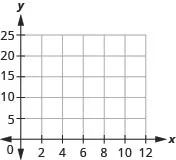Why is only Quadrant I needed?

 Age $xx$ Weight $yy$ $(x,y)(x,y)$ 0 7 (0, 7) 2 11 (2, 11) 4 15 (4, 15) 6 16 (6, 16) 8 19 (8, 19) 10 20 (10, 20) 12 21 (12, 21)
50.

Weight of a child. Latresha recorded her son’s height and weight every year. His height, in inches, and weight, in pounds, are listed in the table below, and shown as an ordered pair in the third column.

Plot the points on a coordinate plane.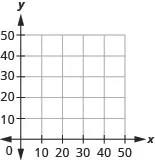Why is only Quadrant I needed?

 Height $xx$ Weight $yy$ $(x,y)(x,y)$ 28 22 (28, 22) 31 27 (31, 27) 33 33 (33, 33) 37 35 (37, 35) 40 41 (40, 41) 42 45 (42, 45)

#### Writing Exercises

51.

Explain in words how you plot the point $(4,−2)(4,−2)$ in a rectangular coordinate system.

52.

How do you determine if an ordered pair is a solution to a given equation?

53.

Is the point $(−3,0)(−3,0)$ on the x-axis or y-axis? How do you know?

54.

Is the point $(0,8)(0,8)$ on the x-axis or y-axis? How do you know?

#### Self Check

After completing the exercises, use this checklist to evaluate your mastery of the objectives of this section.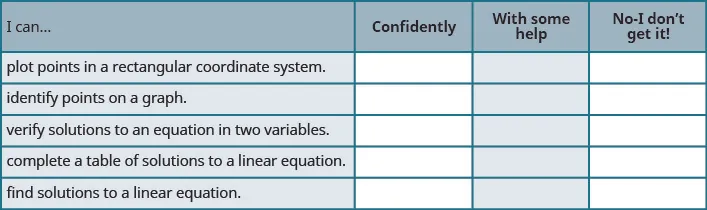If most of your checks were:

…confidently. Congratulations! You have achieved the objectives in this section. Reflect on the study skills you used so that you can continue to use them. What did you do to become confident of your ability to do these things? Be specific.

…with some help. This must be addressed quickly because topics you do not master become potholes in your road to success. In math every topic builds upon previous work. It is important to make sure you have a strong foundation before you move on. Whom can you ask for help? Your fellow classmates and instructor are good resources. Is there a place on campus where math tutors are available? Can your study skills be improved?

…no, I don’t get it. This is a warning sign and you must not ignore it. You should get help right away or you will quickly be overwhelmed. See your instructor as soon as you can to discuss your situation. Together you can come up with a plan to get you the help you need.

Order a print copy

As an Amazon Associate we earn from qualifying purchases.